### IMO Shortlist 2007 problem G4

Kvaliteta:
Avg: 0,0
Težina:
Avg: 7,0
Consider five points$A$,$B$,$C$,$D$ and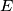$E$ such that$ABCD$ is a parallelogram and$BCED$ is a cyclic quadrilateral. Let$\ell$ be a line passing through$A$. Suppose that$\ell$ intersects the interior of the segment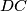$DC$ at$F$ and intersects line$BC$ at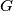$G$. Suppose also that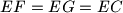$EF = EG = EC$. Prove that$\ell$ is the bisector of angle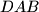$DAB$.

Author: Charles Leytem, Luxembourg
Izvor: Međunarodna matematička olimpijada, shortlist 2007